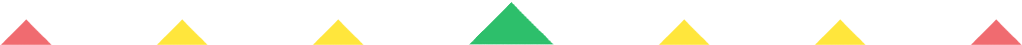Player 1
vs
Player 2
0:00
Opponent history
x
xCorrect
0
Wrong
0
Correct!
Wrong!
Help Sheet for

• 0 + 2 = 2
• 1 + 2 = 3
• 2 + 2 = 4
• 3 + 2 = 5
• 4 + 2 = 6
• 5 + 2 = 7
• 6 + 2 = 8
• 7 + 2 = 9
• 8 + 2 = 10